Geometry symbols

Geometry and trigonometry are branches of mathematics concerned with geometrical figures and angles of triangles. The following list documents some of the most notable symbols in these topics, along with each symbol’s usage and meaning.

For readability purpose, these symbols are categorized by their function into tables. Other comprehensive lists of math symbols — as categorized by subject and type — can be also found in the relevant pages below (or in the navigational panel).

In geometry, points and lines form the foundation of more complex geometrical figures such as triangles, circles, quadrilaterals and polygons. The following table documents some of the most notable symbols related to these — along with each symbol’s meaning and example.

Table of symbols in geometry:

Symbol Symbol Name Meaning / definition Example
angle formed by two rays ∠ABC = 30°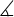measured angleABC = 30°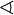spherical angleAOB = 30°
right angle = 90° α = 90°
° degree 1 turn = 360° α = 60°
deg degree 1 turn = 360deg α = 60deg
prime arcminute, 1° = 60′ α = 60°59′
double prime arcsecond, 1′ = 60″ α = 60°59′59″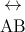line infinite line
AB line segment line from point A to point B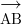ray line that start from point A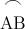arc arc from point A to point B= 60°
perpendicular perpendicular lines (90° angle) ACBC
parallel parallel lines ABCD
congruent to equivalence of geometric shapes and size ∆ABC ≅ ∆XYZ
~ similarity same shapes, not same size ∆ABC ~ ∆XYZ
Δ triangle triangle shape ΔABC ≅ ΔBCD
|x-y| distance distance between points x and y | x-y | = 5
π pi constant π = 3.141592654...

is the ratio between the circumference and diameter of a circle

c = πd = 2⋅πr

Algebra symbols »

Currently, we have around 1977 calculators, conversion tables and usefull online tools and software features for students, teaching and teachers, designers and simply for everyone.